#### Varieties

For a given field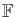and positive integer, the-dimensional affine space overis the set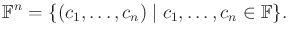(4.51)

For our purposes in this section, an affine space can be considered as a vector space (for an exact definition, see ). Thus,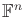is like a vector version of the scalar field. Familiar examples of this are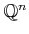,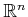, and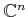.

A polynomial in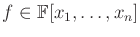can be converted into a function,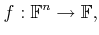(4.52)

by substituting elements offor each variable and evaluating the expression using the field operations. This can be written as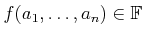, in which each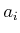denotes an element ofthat is substituted for the variable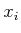.

We now arrive at an interesting question. For a given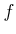, what are the elements ofsuch that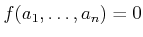? We could also ask the question for some nonzero element, but notice that this is not necessary because the polynomial may be redefined to formulate the question using 0. For example, what are the elements of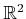such that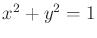? This familiar equation for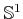can be reformulated to yield: What are the elements ofsuch that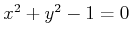?

Letbe a field and let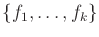be a set of polynomials in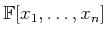. The setfor all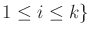(4.53)

is called the (affine) variety defined by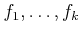. One interesting fact is that unions and intersections of varieties are varieties. Therefore, they behave like the semi-algebraic sets from Section 3.1.2, but for varieties only equality constraints are allowed. Consider the varieties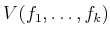and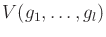. Their intersection is given by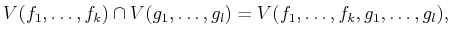(4.54)

because each element ofmust produce a 0 value for each of the polynomials in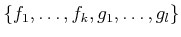.

To obtain unions, the polynomials simply need to be multiplied. For example, consider the varieties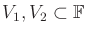defined as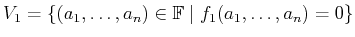(4.55)

and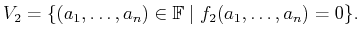(4.56)

The set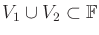is obtained by forming the polynomial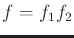. Note thatif either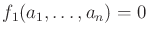or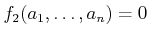. Therefore,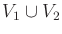is a variety. The varieties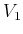andwere defined using a single polynomial, but the same idea applies to any variety. All pairs of the form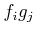must appear in the argument of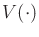if there are multiple polynomials.

Steven M LaValle 2020-08-14